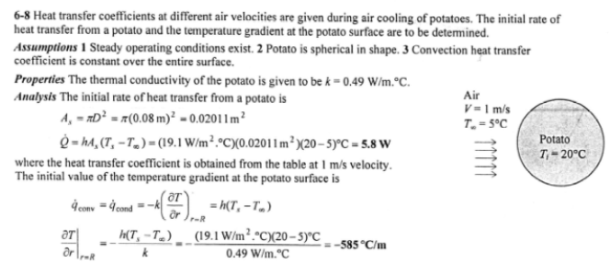heat and mass transfer problems engineering equations heat and mass transfer school homework engineering heat and mass transfer formulas heat and mass transfer solutions to heat and mass transfer problems full solution engineering problem solution heat and mass transfer math problems engineering equations heat and mass transfer school homework engineering solutions to heat and mass transfer formulas heat problems mass problem solutions to transfer problems full solution heat and mass transfer
heat and mass transfer problems engineering equations heat and mass transfer school homework engineering heat and mass transfer formulas heat and mass transfer solutions to heat and mass transfer problems full solution engineering problem solution heat and mass transfer math problems engineering equations heat and mass transfer school homework engineering solutions to heat and mass transfer formulas heat problems mass problem solutions to transfer problems full solution heat and mass transfer
Highalphabet Home Page heat and mass transfer problem solutions Heat and Mass Transfer Page
During air cooling of potatoes, the heat transfer coefficient for combined convection, radiation, and evaporation is determined experimentally to be as shown: Air Velocity, m/s: 0.66, 1.00, 1.36, 1.73; Heat transfer Coefficient, W/m^2K: 14.0, 19.1, 20.2, 24.4 . Consider an 8-cm-diameter potato initially at 20C with a thermal conductivity of 0.49 W/mK. Potatoes are cooled by refrigerated air at 5 C at a velocity of 1 m/s. Determine the initial rate of heat transfer from a potato, and the initial value of the temperature gradient in the potato at the surface.During air cooling of potatoes, the heat transfer coefficient for combined convection, radiation, and evaporation is determined experimentally to be as shown: Air Velocity, m/s: 0.66, 1.00, 1.36, 1.73; Heat transfer Coefficient, W/m^2K: 14.0, 19.1, 20.2, 24.4 . Consider an 8-cm-diameter potato initially at 20C with a thermal conductivity of 0.49 W/mK. Potatoes are cooled by refrigerated air at 5 C at a velocity of 1 m/s. Determine the initial rate of heat transfer from a potato, and the initial value of the temperature gradient in the potato at the surface.